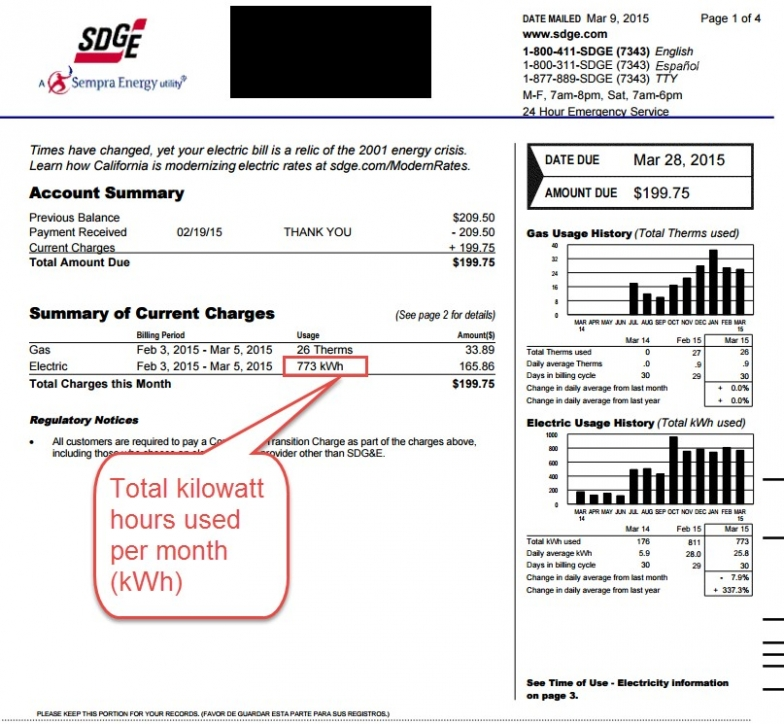## What if my home is notably larger or smaller than the “average” home in my region?

For the great majority of homeowners, the average price should be sufficient. If your home is a lot larger or smaller than “average”, (three bedrooms and two bathrooms) use the following steps estimate how much the cost for your home would be:

1) Get your power bill, and determine how many kilowatt hours you used in the last month.

2) Use the following formula for a rough estimate of what size solar system you would need, in kilowatts, to estimate your home’s appropriate-sized solar system:

KW solar system size required = (Total # of kWh used)/30/7

In the below example:

KW solar system size required = 773 kWh / 30 days in a month / 7 hours per day of equivalent sunlight = 3.68 KW system required.

3) Multiply the number of Kilowatts by both the before and after-incentive cost in your area:

Approximate average cost of solar for your home:

After incentive solar cost – the actual cost of the solar system to the homeowner, after government and utility incentives and rebates are factored in:

After incentive cost per kilowatt x number of kilowatts required.

Before incentive cost – the cost of solar before any incentives and rebates are applied – this number is NOT your true cost – as the incentives and rebates reduce your costs significantly:

Before incentive cost per kilowatt x number of kilowatts required.

Example:

If the price/kilowatt in your area was: \$2,900 after incentives (so your true cost) and \$4,500 before incentives.

How much you would pay as a homeowner (after incentives and rebates):

\$2,900 per kilowatt x 3.68 kW = Total cost of solar to the homeowner: \$10,672

How much solar panels would be before incentive savings:

\$4,500 per kilowatt x 3.68 kW = Total cost of solar to the homeowner (before incentives and rebates): \$16,560Start typing and press Enter to search